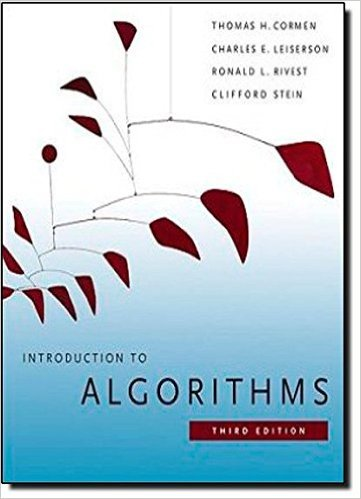×
Get Full Access to Introduction To Algorithms - 3 Edition - Chapter 3 - Problem 3-4
Get Full Access to Introduction To Algorithms - 3 Edition - Chapter 3 - Problem 3-4

×

# Asymptotic notation properties Let f .n/ and g.n/ beISBN: 9780262033848 130

## Solution for problem 3-4 Chapter 3

Introduction to Algorithms | 3rd Edition

• Textbook Solutions
• 2901 Step-by-step solutions solved by professors and subject experts
• Get 24/7 help from StudySoup virtual teaching assistantsIntroduction to Algorithms | 3rd Edition

4 5 1 321 Reviews
18
4
Problem 3-4

Asymptotic notation properties Let f .n/ and g.n/ be asymptotically positive functions. Prove or disprove each of the following conjectures. a. f .n/ D O.g.n// implies g.n/ D O.f .n//. b. f .n/ C g.n/ D .min.f .n/; g.n///. c. f .n/ D O.g.n// implies lg.f .n// D O.lg.g.n///, where lg.g.n// 1 and f .n/ 1 for all sufficiently large n. d. f .n/ D O.g.n// implies 2f .n/ D O 2g.n/ . e. f .n/ D O ..f .n//2/. f. f .n/ D O.g.n// implies g.n/ D .f .n//. g. f .n/ D .f .n=2//. h. f .n/ C o.f .n// D .f .n//.

Step-by-Step Solution:
Step 1 of 3

Step 2 of 3

Step 3 of 3

##### ISBN: 9780262033848

Unlock Textbook Solution